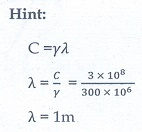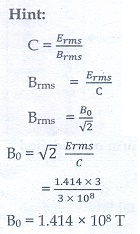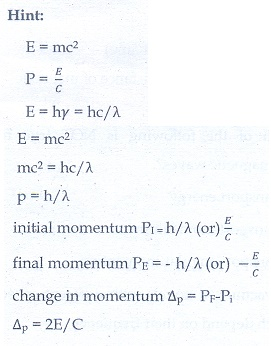# Unit V: Electromagnetic waves - Online Test

Q1. The dimension of 1/µ0ε0 is
Explaination / Solution:Q2. If the amplitude of the magnetic field is 3 × 10−6 T, then amplitude of the electric field for a electromagnetic waves is
Explaination / Solution:Q3. Which of the following electromagnetic radiation is used for viewing objects through fog
Explaination / Solution:
No Explaination.

Q4. Which of the following are false for electromagnetic waves
Explaination / Solution:
No Explaination.

Q5. Consider an oscillator which has a charged particle and oscillates about its mean position with a frequency of 300 MHz. The wavelength of electromagnetic waves produced by this oscillator is
Explaination / Solution:Q6. The electric and the magnetic field, associated with an electromagnetic wave, propagating along X axis can be represented by
Explaination / Solution:
No Explaination.

Q7. In an electromagnetic wave in free space the rms value of the electric field is 3 V m−1. The peak value of the magnetic field is
Explaination / Solution:Q8. During the propagation of electromagnetic waves in a medium:
Explaination / Solution:
No Explaination.

Q9. If the magnetic monopole exists, then which of the Maxwell’s equation to be modified?.
Explaination / Solution:
No Explaination.

Q10. A radiation of energy E falls normally on a perfectly reflecting surface. The momentum transferred to the surface is
Explaination / Solution: